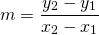# Using Slope and y intercept

Use the formulato find the slope of each line. Use the vertical intercept to complete the equation in the formWorked Example: example slope y intercept

Test out on Slope y intercept

# Using Two Points

Use the formulato find the slope of each line.

Now substitute theandcoordinates of one of the points to find the value. Type in your formula in the formExample: Example two points

# Using One Point and the Slope

The formula